# Work + system of equations - math problems

Work Problems are word problems that involve different people doing work together but at different rates. Another problem that involves pipes filling up a tank. Another name for these problems is working together word problems.

We suggest solving working together word problems with the proportion. If a task can be completed in t hours, then 1/t of the task can be completed In one hour.

#### Number of problems found: 25

• Sow barley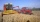Farmers wanted to sow barley within 13 days. Due to the excellent weather, they managed to exceed the daily plan of sowing by 2 ha and therefore finished sow grain in 12 days. How many hectares of land did they sow with barley?
• Working together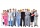Two people will do the work in 12 days. They worked together for 8 days. Then only one worked for 10 days. How many days would each of them do the work if he worked alone?
• Flowerbed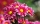Ondra digs the bed in 20 minutes. Cuba in 30 minutes. For how long do they dig together?
• Tractors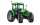Wheel and belt tractors each day plow 8 ha of fields. For plow 76 hectares, the wheel would have to work for 12 days and 8 days with the belt tractor together. How many hectares do each day of each tractor?
• Two diggersTwo diggers should dig a ditch. If each of them worked just one-third of the time that the other digger needs, they'd dig up a 13/18 ditch together. Find the ratio of the performance of this two diggers.
• Two pumpsPump A takes twice as long to fill a tank than B . Together they take 2 hours to fill the tank. How long does each one take to fill the tank by itself?
• Tractors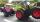Two tractors plow the field in 4 hours together. If the first tractor plow half of the field and then the second tractor completed the job, it would take 9 hours. How many hours does the field plow for each tractor separately?
• Two workersTwo workers should fulfill certain task together for 5 days. If the first worker increased their performance twice and second twice fell, it took them just four days. For how many days would handle the entire task first worker himself?
• PotatoesDaniela and Michael would jointly dug potatoes for 7.5 hours. But if Daniela was working alone she would take 2.5 hours more as if he were working with Michael. Determine how much for the work done by Michael himself and how much Daniela herself.
• Three piles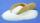100 kg of sugar we divide into three piles. The first pile is small. If we added to second 2 kg of sugar it would have 25% more sugar than the first pile. If we added to third pile 3 kg of sugar it would have 20% more sugar than the 2nd pile. How many kil
• Two pipes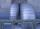One pipe fill one-fifth volume 20 minutes before by second one. The two pipes together will fill the tank in two hours. How long is will fill tank each pipe separately?
• Two workers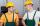Two workers work together and perform the job in 12 hours. If one has done half the work and then second worker next half of work it will take 25 hours. How long would it take job them separately?
• WorkersTen workers must pave road street for 22 working days. After four days were for speeding up work added two more workers. a) After how many work days now workers completes the paved road? b) How many working days it took a total paved road?
• Tank 9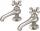The tank with volume V is filled with one pump for three hours and by second pump for 5 hours. When both pumps will run simultaneously calculate: a) how much of the total volume of the tank is filled in one hour b) for how long is the tank full
• Water pool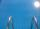Pool with volume 990hl completely filled, if water flows by one tap 8 hours and by second tap 6 hours. First tap give 10hl more than second per hour. How many hl flows in each of them in an hour?
• James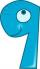James paints a fence. If every day instead of 14 planks painted 16 would be done on one day earlier. How many of planks has a fence altogether?
• WorkhorsesA team of colleagues done the work in 20 days. After adding 2 colleagues from the beginning the work was completed in 15 days. How many members has the colleague team?
• Two workers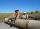Two workers carry will do certain work for 12 days. After 8 days of working was one removed, and then the other finished the job alone in 10 days. For how many days would do this work alone each worker?
• Three workers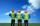Three workers A, B, C have to work on a specific task. Workers A and B completed whole task in 14 days, B together with C for 23 days, A together with C for 13 days. How long it would take to complete the task every one of them alone? How long it would ta
• Two valves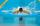Water fill the pool by two valves for 11 days. After 7 days the first valve was stopped and the second valve fill pool for 7 days. How many days took to fill the pool each of the valves individually?

Do you have an interesting mathematical word problem that you can't solve it? Submit a math problem, and we can try to solve it.

We will send a solution to your e-mail address. Solved examples are also published here. Please enter the e-mail correctly and check whether you don't have a full mailbox.

Please do not submit problems from current active competitions such as Mathematical Olympiad, correspondence seminars etc...

Do you have a system of equations and looking for calculator system of linear equations?